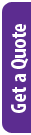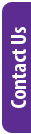## Details

Performing regressions is a powerful method to help you understand the relationships between your variables, which makes it a must for anyone working with data. Thankfully, we can use the Python language to make this important task much easier and more efficient.

In this course you will learn to:

• Perform regressions using Python
• Analyse the strength of correlations
• Set conditional expectations
• Analyse the regression line
• Employ linear models in real-life scenarios
• Understand counfounding
• Perform Lease Squares Estimates
• Understand association vs causation

### Delivery Methods

Leading Training is focusing on providing virtual training courses for the foreseeable future and will only consider in-person and classroom training on request, with a required minimum group size of four delegates. We remain committed to offering training that is fast, focused and effective.

Delivery Method Duration Price (excl. VAT)
Fulltime 4 Days R 11,596.00
Webinar 4 Days R 9,400.00

### Brochure:

Information may change without notice.

## Audience

• Data scientists
• Programmers
• Engineers
• Scientists

## Pre-Requisites

Leading Training's Introduction to R Programming or equivalent knowledge

## Course Outline / Curriculum

Regression

• Case study: is height hereditary?
• The correlation coefficient
• Sample correlation is a random variable
• Correlation is not always a useful summary
• Conditional expectations
• The regression line
• Regression improves precision
• Variance explained
• Warning: there are two regression lines
• Exercises

Linear models

• Case study: Moneyball
• Sabermetics
• Baseball basics
• No awards for BB
• Base on balls or stolen bases?
• Regression applied to baseball statistics
• Confounding
• Understanding confounding through stratification
• Multivariate regression
• Least squares estimates
• Interpreting linear models
• Least Squares Estimates (LSE)
• The lm function
• LSE are random variables
• Predicted values are random variables
• Exercises
• Linear regression in the tidyverse
• The broom package
• Exercises
• Case study: Moneyball (continued)
• Adding salary and position information
• Picking nine players
• The regression fallacy
• Measurement error models
• Exercises

Association is not causation

• Spurious correlation
• Outliers
• Reversing cause and effect
• Confounders
• Confounding explained graphically
• Average after stratifying